# Godel’s Completeness and Incompleteness Theorems

Followup to: Standard and Nonstandard Numbers

So… last time you claimed that using first-order axioms to rule out the existence of nonstandard numbers—other chains of numbers besides the ‘standard’ numbers starting at 0 - was forever and truly impossible, even unto a superintelligence, no matter how clever the first-order logic used, even if you came up with an entirely different way of axiomatizing the numbers.

“Right.”

How could you, in your finiteness, possibly know that?

“Have you heard of Godel’s Incompleteness Theorem?”

Of course! Godel’s Theorem says that for every consistent mathematical system, there are statements which are true within that system, which can’t be proven within the system itself. Godel came up with a way to encode theorems and proofs as numbers, and wrote a purely numerical formula to detect whether a proof obeyed proper logical syntax. The basic trick was to use prime factorization to encode lists; for example, the ordered list <3, 7, 1, 4> could be uniquely encoded as:

23 * 37 * 51 * 74

And since prime factorizations are unique, and prime powers don’t mix, you could inspect this single number, 210,039,480, and get the unique ordered list <3, 7, 1, 4> back out. From there, going to an encoding for logical formulas was easy; for example, you could use the 2 prefix for NOT and the 3 prefix for AND and get, for any formulas Φ and Ψ encoded by the numbers #Φ and #Ψ:

¬Φ = 22 * 3

Φ ∧ Ψ = 23 * 3 * 5

It was then possible, by dint of crazy amounts of work, for Godel to come up with a gigantic formula of Peano Arithmetic [](p, c) meaning, ‘P encodes a valid logical proof using first-order Peano axioms of C’, from which directly followed the formula []c, meaning, ‘There exists a number P such that P encodes a proof of C’ or just ‘C is provable in Peano arithmetic.’

Godel then put in some further clever work to invent statements which referred to themselves, by having them contain sub-recipes that would reproduce the entire statement when manipulated by another formula.

And then Godel’s Statement encodes the statement, ‘There does not exist any number P such that P encodes a proof of (this statement) in Peano arithmetic’ or in simpler terms ‘I am not provable in Peano arithmetic’. If we assume first-order arithmetic is consistent and sound, then no proof of this statement within first-order arithmetic exists, which means the statement is true but can’t be proven within the system. That’s Godel’s Theorem.

“Er… no.”

No?

“No. I’ve heard rumors that Godel’s Incompleteness Theorem is horribly misunderstood in your Everett branch. Have you heard of Godel’s Completeness Theorem?”

Is that a thing?

Yes! Godel’s Completeness Theorem says that, for any collection of first-order statements, every semantic implication of those statements is syntactically provable within first-order logic. If something is a genuine implication of a collection of first-order statements—if it actually does follow, in the models pinned down by those statements—then you can prove it, within first-order logic, using only the syntactical rules of proof, from those axioms.”

I don’t see how that could possibly be true at the same time as Godel’s Incompleteness Theorem. The Completeness Theorem and Incompleteness Theorem seem to say diametrically opposite things. Godel’s Statement is implied by the axioms of first-order arithmetic—that is, we can see it’s true using our own mathematical reasoning -

“Wrong.”

What? I mean, I understand we can’t prove it within Peano arithmetic, but from outside the system we can see that -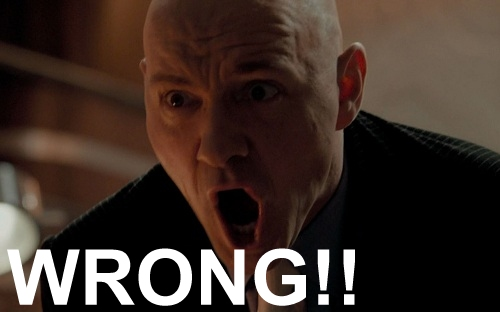All right, explain.

“Basically, you just committed the equivalent of saying, ‘If all kittens are little, and some little things are innocent, then some kittens are innocent.’ There are universes—logical models—where it so happens that the premises are true and the conclusion also happens to be true:”“But there are also valid models of the premises where the conclusion is false:”“If you, yourself, happened to live in a universe like the first one—if, in your mind, you were only thinking about a universe like that—then you might mistakenly think that you’d proven the conclusion. But your statement is not logically valid, the conclusion is not true in every universe where the premises are true. It’s like saying, ‘All apples are plants. All fruits are plants. Therefore all apples are fruits.’ Both the premises and the conclusions happen to be true in this universe, but it’s not valid logic.”

Okay, so how does this invalidate my previous explanation of Godel’s Theorem?

“Because of the non-standard models of first-order arithmetic. First-order arithmetic narrows things down a lot—it rules out 3-loops of nonstandard numbers, for example, and mandates that every model contain the number 17 - but it doesn’t pin down a single model. There’s still the possibility of infinite-in-both-directions chains coming after the ‘standard’ chain that starts with 0. Maybe you have just the standard numbers in mind, but that’s not the only possible model of first-order arithmetic.”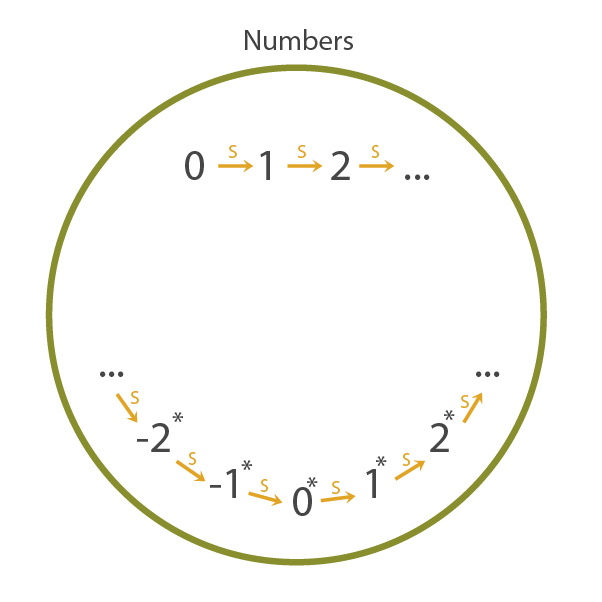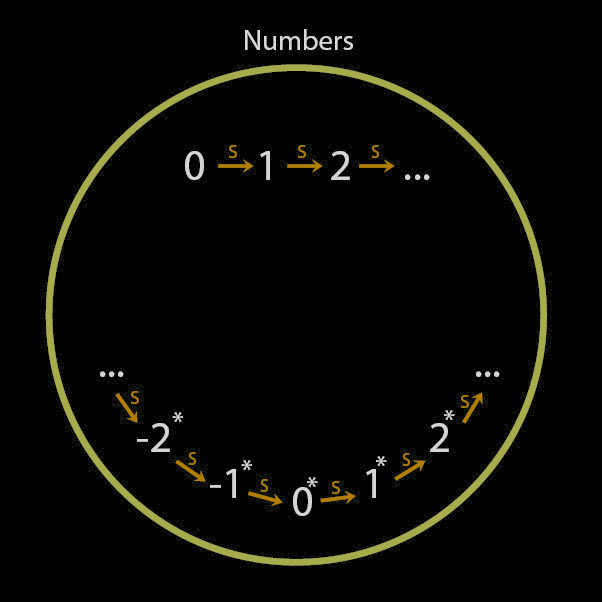So?

“So in some of those other models, there are nonstandard numbers which—according to Godel’s arithmetical formula for encodes-a-proof—are ‘nonstandard proofs’ of Godel’s Statement. I mean, they’re not what we would call actual proofs. An actual proof would have a standard number corresponding to it. A nonstandard proof might look like… well, it’s hard to envision, but it might be something like, ‘Godel’s statement is true, because not-not-Godel’s statement, because not-not-not-not-Godel’s statement’, and so on going backward forever, every step of the proof being valid, because nonstandard numbers have an infinite number of predecessors.”

And there’s no way to say, ‘You can’t have an infinite number of derivations in a proof’?

“Not in first-order logic. If you could say that, you could rule out numbers with infinite numbers of predecessors, meaning that you could rule out all infinite-in-both-directions chains, and hence rule out all nonstandard numbers. And then the only remaining model would be the standard numbers. And then Godel’s Statement would be a semantic implication of those axioms; there would exist no number encoding a proof of Godel’s Statement in any model which obeyed the axioms of first-order arithmetic. And then, by Godel’s Completeness Theorem, we could prove Godel’s Statement from those axioms using first-order syntax. Because every genuinely valid implication of any collection of first-order axioms—every first-order statement that actually does follow, in every possible model where the premises are true—can always be proven, from those axioms, in first-order logic. Thus, by the combination of Godel’s Incompleteness Theorem and Godel’s Completeness Theorem, we see that there’s no way to uniquely pin down the natural numbers using first-order logic. QED.”

Whoa. So everyone in the human-superiority crowd gloating about how they’re superior to mere machines and formal systems, because they can see that Godel’s Statement is true just by their sacred and mysterious mathematical intuition...

″...Is actually committing a horrendous logical fallacy of the sort that no cleanly designed AI could ever be tricked into, yes. Godel’s Statement doesn’t actually follow from the first-order axiomatization of Peano arithmetic! There are models where all the first-order axioms are true, and yet Godel’s Statement is false! The standard misunderstanding of Godel’s Statement is something like the situation as it obtains in second-order logic, where there’s no equivalent of Godel’s Completeness Theorem. But people in the human-superiority crowd usually don’t attach that disclaimer—they usually present arithmetic using the first-order version, when they’re explaining what it is that they can see that a formal system can’t. It’s safe to say that most of them are inadvertently illustrating the irrational overconfidence of humans jumping to conclusions, even though there’s a less stupid version of the same argument which invokes second-order logic.”

Nice. But still… that proof you’ve shown me seems like a rather circuitous way of showing that you can’t ever rule out infinite chains, especially since I don’t see why Godel’s Completeness Theorem should be true.

“Well… an equivalent way of stating Godel’s Completeness Theorem is that every syntactically consistent set of first-order axioms—that is, every set of first-order axioms such that you cannot syntactically prove a contradiction from them using first-order logic—has at least one semantic model. The proof proceeds by trying to adjoin statements saying P or ~P for every first-order formula P, at least one of which must be possible to adjoin while leaving the expanded theory syntactically consistent—”

Hold on. Is there some more constructive way of seeing why a non-standard model has to exist?

“Mm… you could invoke the Compactness Theorem for first-order logic. The Compactness Theorem says that if a collection of first-order statements has no model, some finite subset of those statements is also semantically unrealizable. In other words, if a collection of first-order statements—even an infinite collection—is unrealizable in the sense that no possible mathematical model fits all of those premises, then there must be some finite subset of premises which are also unrealizable. Or modus ponens to modus tollens, if all finite subsets of a collection of axioms have at least one model, then the whole infinite collection of axioms has at least one model.”

Ah, and can you explain why the Compactness Theorem should be true?

No.

I see.

“But at least it’s simpler than the Completeness Theorem, and from the Compactness Theorem, the inability of first-order arithmetic to pin down a standard model of numbers follows immediately. Suppose we take first-order arithmetic, and adjoin an axiom which says, ‘There exists a number greater than 0.’ Since there does in fact exist a number, 1, which is greater than 0, first-order arithmetic plus this new axiom should be semantically okay—it should have a model if any model of first-order arithmetic ever existed in the first place. Now let’s adjoin a new constant symbol c to the language, i.e., c is a constant symbol referring to a single object across all statements where it appears, the way 0 is a constant symbol and an axiom then identifies 0 as the object which is not the successor of any object. Then we start adjoining axioms saying ‘c is greater than X’, where X is some concretely specified number like 0, 1, 17, 2256, and so on. In fact, suppose we adjoin an infinite series of such statements, one for every number:”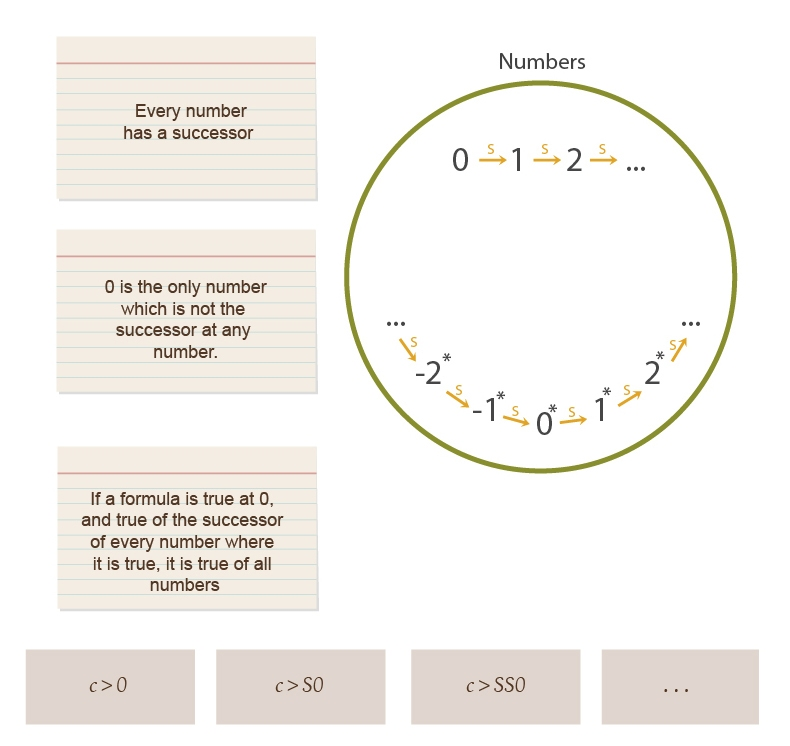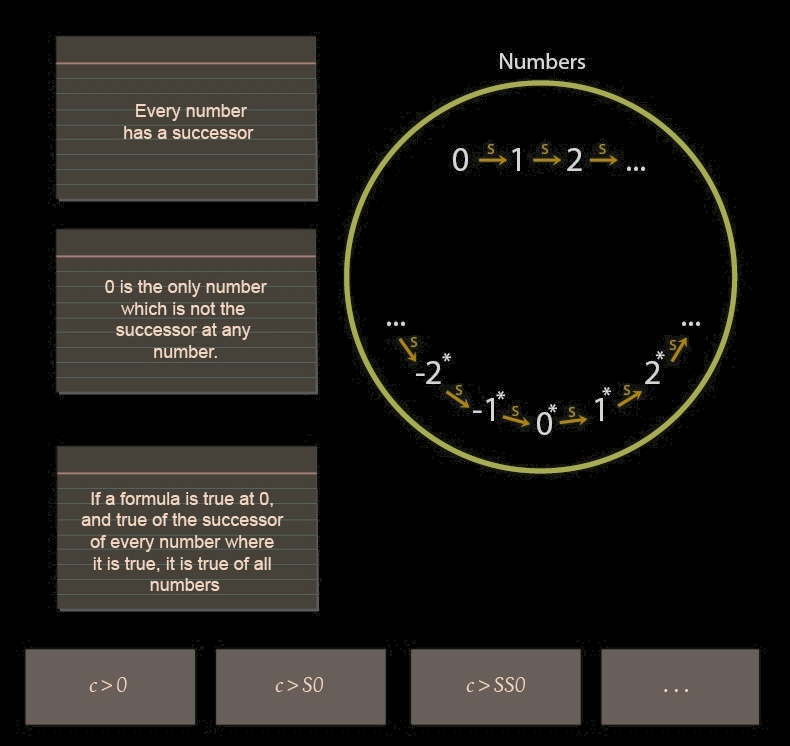Wait, so this new theory is saying that there exists a number c which is larger than every number?

“No, the infinite schema says that there exists a number c which is larger than any standard number.”

I see, so this new theory forces a nonstandard model of arithmetic.

“Right. It rules out only the standard model. And the Compactness Theorem says this new theory is still semantically realizable—it has some model, just not the standard one.”

Why?

“Because any finite subcollection of the new theory’s axioms, can only use a finite number of the extra axioms. Suppose the largest extra axiom you used was ‘c is larger than 2256’. In the standard model, there certainly exists a number 2256+1 with which c could be consistently identified. So the standard numbers must be a model of that collection of axioms, and thus that finite subset of axioms must be semantically realizable. Thus by the Compactness Theorem, the full, infinite axiom system must also be semantically realizable; it must have at least one model. Now, adding axioms never increases the number of compatible models of an axiom system—each additional axiom can only filter out models, not add models which are incompatible with the other axioms. So this new model of the larger axiom system—containing a number which is greater than 0, greater than 1, and greater than every other ‘standard’ number—must also be a model of first-order Peano arithmetic. That’s a relatively simpler proof that first-order arithmetic—in fact, any first-order axiomatization of arithmetic—has nonstandard models.”

Huh… I can’t quite say that seems obvious, because the Compactness Theorem doesn’t feel obvious; but at least it seems more specific than trying to prove it using Godel’s Theorem.

“A similar construction to the one we used above—adding an infinite series of axioms saying that a thingy is even larger—shows that if a first-order theory has models of unboundedly large finite size, then it has at least one infinite model. To put it even more alarmingly, there’s no way to characterize the property of finiteness in first-order logic! You can have a first-order theory which characterizes models of cardinality 3 - just say that there exist x, y, and z which are not equal to each other, but with all objects being equal to x or y or z. But there’s no first-order theory which characterizes the property of finiteness in the sense that all finite models fit the theory, and no infinite model fits the theory. A first-order theory either limits the size of models to some particular upper bound, or it has infinitely large models.”

So you can’t even say, ‘x is finite’, without using second-order logic? Just forming the concept of infinity and distinguishing it from finiteness requires second-order logic?

“Correct, for pretty much exactly the same reason you can’t say ‘x is only a finite number of successors away from 0’. You can say, ‘x is less than a googolplex’ in first-order logic, but not, in full generality, ‘x is finite’. In fact there’s an even worse theorem, the Lowenheim-Skolem theorem, which roughly says that if a first-order theory has any infinite model, it has models of all possible infinite cardinalities. There are uncountable models of first-order Peano arithmetic. There are countable models of first-order real arithmetic—countable models of any attempt to axiomatize the real numbers in first-order logic. There are countable models of Zermelo-Frankel set theory.”

How could you possibly have a countable model of the real numbers? Didn’t Cantor prove that the real numbers were uncountable? Wait, let me guess, Cantor implicitly used second-order logic somehow.

“It follows from the Lowenheim-Skolem theorem that he must’ve. Let’s take Cantor’s proof as showing that you can’t map every set of integers onto a distinct integer—that is, the powerset of integers is larger than the set of integers. The Diagonal Argument is that if you show me a mapping like that, I can take the set which contains 0 if and only if 0 is not in the set mapped to the integer 0, contains 1 if and only if 1 is not in the set mapped to the integer 1, and so on. That gives you a set of integers that no integer maps to.”

You know, when I was very young indeed, I thought I’d found a counterexample to Cantor’s argument. Just take the base-2 integers − 1=‘1’, 2=’10′, 3=‘11’, 4=‘100’, 5=‘101’, and so on, and let each integer correspond to a set in the obvious way, keeping in mind that I was also young enough to think the integers started at 1:

 1 10 11 100 101 110 111 1000 1001 {1} {2} {2, 1} {3} {3, 1} {3, 2} {3, 2, 1} {4} {4, 1}

Clearly, every set of integers would map onto a unique integer this way.

“Heh.”

Yeah, I thought I was going to be famous.

“How’d you realize you were wrong?”

After an embarrassingly long interval, it occurred to me to actually try applying Cantor’s Diagonal Argument to my own construction. Since 1 is in {1} and 2 is in {2}, they wouldn’t be in the resulting set, but 3, 4, 5 and everything else would be. And of course my construct didn’t have the set {3, 4, 5, …} anywhere in it. I’d mapped all the finite sets of integers onto integers, but none of the infinite sets.

“Indeed.”

I was then tempted to go on arguing that Cantor’s Diagonal Argument was wrong anyhow because it was wrong to have infinite sets of integers. Thankfully, despite my young age, I was self-aware enough to realize I was being tempted to become a mathematical crank—I had also read a book on mathematical cranks by this point—and so I just quietly gave up, which was a valuable life lesson.

“Indeed.”

But how exactly does Cantor’s Diagonal Argument depend on second-order logic? Is it something to do with nonstandard integers?

“Not exactly. What happens is that there’s no way to make a first-order theory contain all subsets of an infinite set; there’s no way to talk about the powerset of the integers. Let’s illustrate using a finite metaphor. Suppose you have the axiom “All kittens are innocent.” One model of that axiom might contain five kittens, another model might contain six kittens.”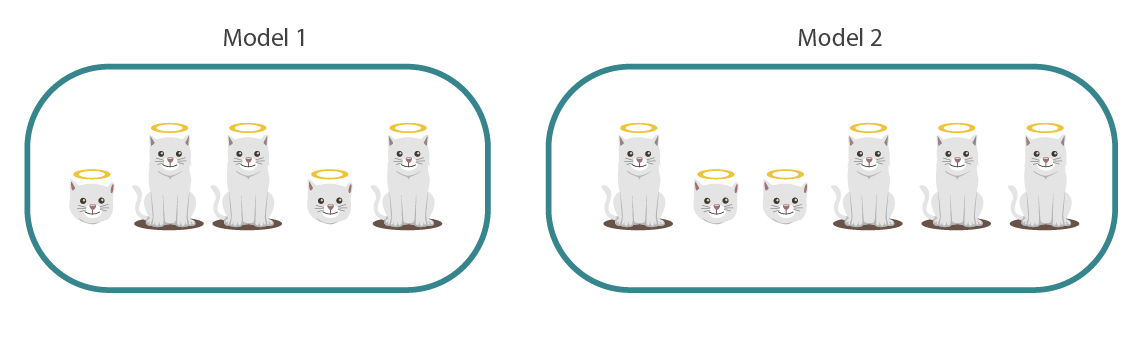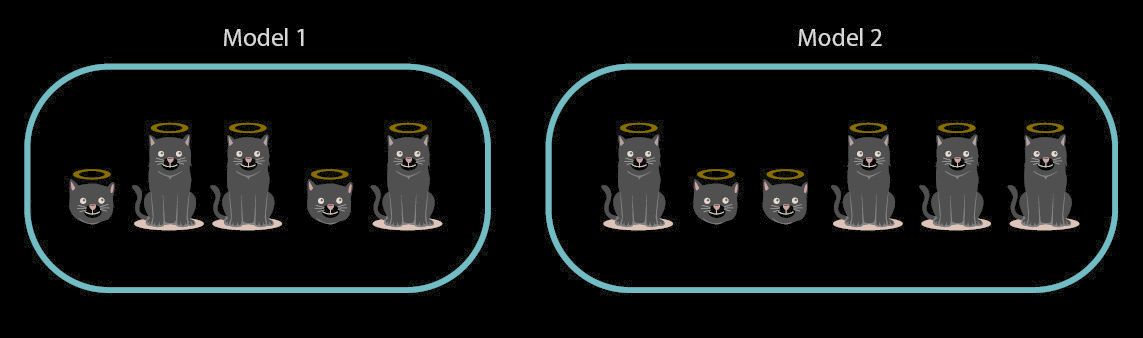“In a second-order logic, you can talk about all possible collections of kittens—in fact, it’s built into the syntax of the language when you quantify over all properties.”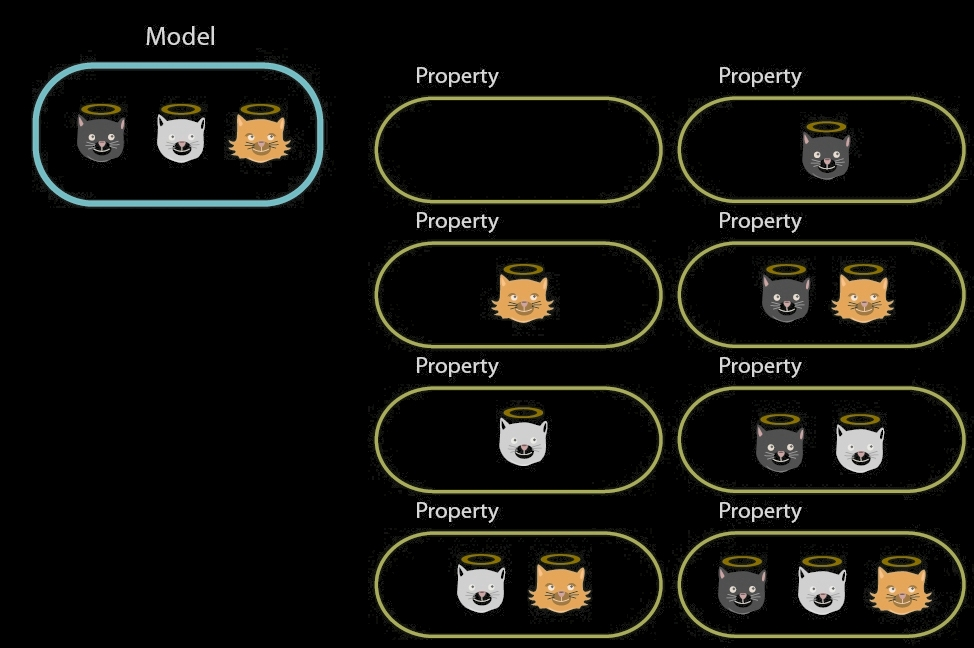“In a first-order set theory, there are some subsets of kittens whose existence is provable, but others might be missing.”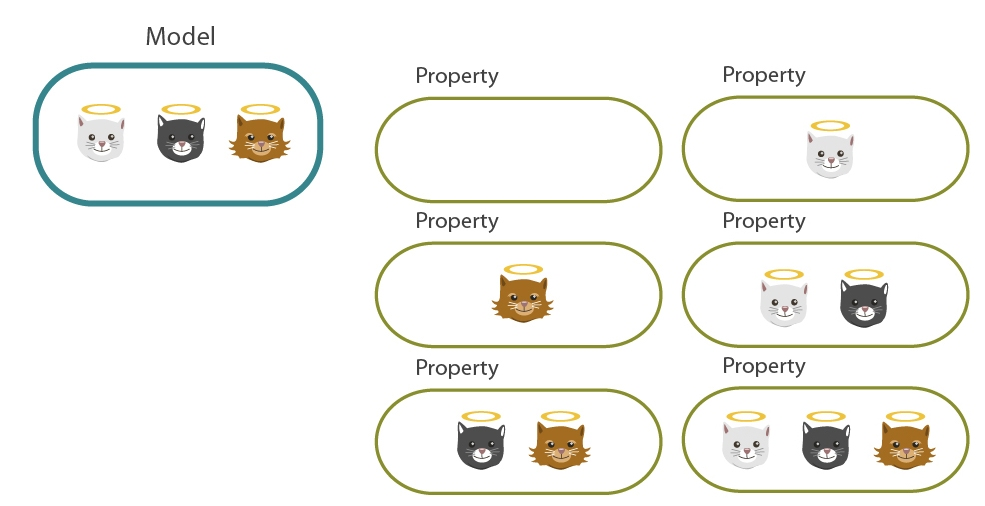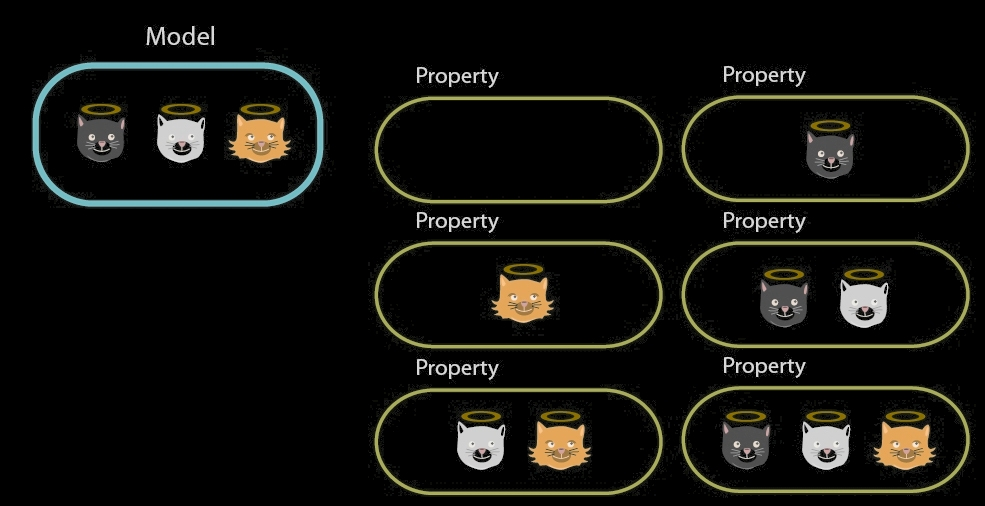“Though that image is only metaphorical, since you can prove the existence of all the finite subsets. Just imagine that’s an infinite number of kittens we’re talking about up there.”

And there’s no way to say that all possible subsets exist?

“Not in first-order logic, just like there’s no way to say that you want as few natural numbers as possible. Let’s look at it from the standpoint of first-order set theory. The Axiom of Powerset says:”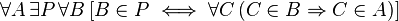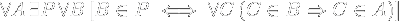Okay, so that says, for every set A, there exists a set P which is the power set of all subsets of A, so that for every set B, B is inside the powerset P if and only if every element of B is an element of A. Any set which contains only elements from A, will be inside the powerset of A. Right?

“Almost. There’s just one thing wrong in that explanation—the word ‘all’ when you say ‘all subsets’. The Powerset Axiom says that for any collection of elements from A, if a set B happens to exist which embodies that collection, that set B is inside the powerset P of A. There’s no way of saying, within a first-order logical theory, that a set exists for every possible collection of A’s elements. There may be some sub-collections of A whose existence you can prove. But other sub-collections of A will happen to exist as sets inside some models, but not exist in others.”

So in the same way that first-order Peano arithmetic suffers from mysterious extra numbers, first-order set theory suffers from mysterious missing subsets.“Precisely. A first-order set theory might happen to be missing the particular infinite set corresponding to, oh, say, {3, 8, 17, 22, 28, …} where the ‘...’ is an infinite list of random numbers with no compact way of specifying them. If there’s a compact way of specifying a set—if there’s a finite formula that describes it—you can often prove it exists. But most infinite sets won’t have any finite specification. It’s precisely the claim to generalize over all possible collections that characterizes second-order logic. So it’s trivial to say in a second-order set theory that all subsets exist. You would just say that for any set A, for any possible predicate P, there exists a set B which contains x iff x in A and Px.”

I guess that torpedoes my clever idea about using first-order set theory to uniquely characterize the standard numbers by first asserting that there exists a set containing at least the standard numbers, and then talking about the smallest subset which obeys the Peano axioms.

“Right. When you talk about the numbers using first-order set theory, if there are extra numbers inside your set of numbers, the subset containing just the standard numbers must be missing from the powerset of that set. Otherwise you could find the smallest subset inside the powerset such that it contained 0 and contained the successor of every number it contained.”

Hm. So then what exactly goes wrong with Cantor’s Diagonal Argument?

“Cantor’s Diagonal Argument uses the idea of a mapping between integers and sets of integers. In set theory, each mapping would itself be a set—in fact there would be a set of all mapping sets:”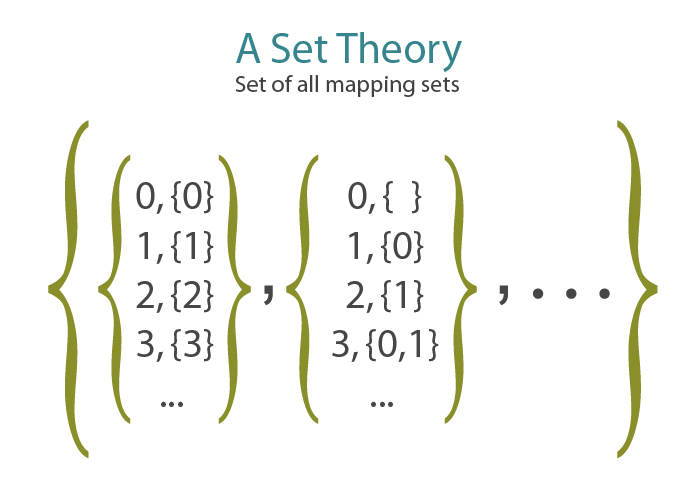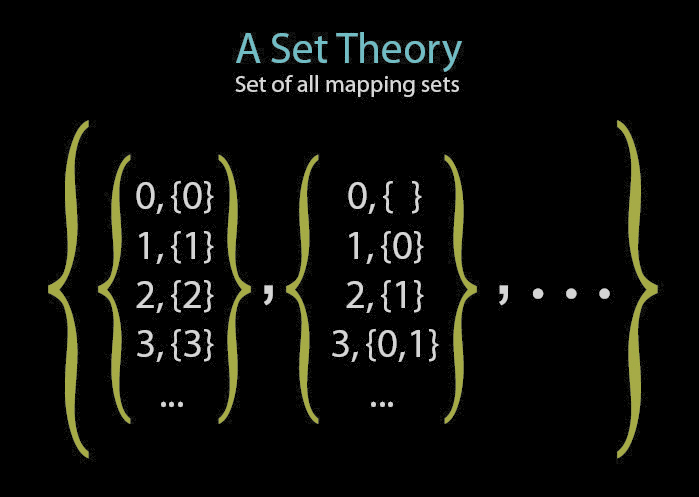“There’s no way to first-order assert the existence of every possible mapping that we can imagine from outside. So a first-order version of the Diagonal Argument would show that in any particular model, for any mapping that existed in the model from integers to sets of integers, the model would also contain a diagonalized set of integers that wasn’t in that mapping. This doesn’t mean that we couldn’t count all the sets of integers which existed in the model. The model could have so many ‘missing’ sets of integers that the remaining sets were denumerable. But then some mappings from integers to sets would also be missing, and in particular, the ‘complete’ mapping we can imagine from outside would be missing. And for every mapping that was in the model, the Diagonal Argument would construct a set of integers that wasn’t in the mapping. On the outside, we would see a possible mapping from integers to sets—but that mapping wouldn’t exist inside the model as a set. It takes a logic-of-collections to say that all possible integer-collections exist as sets, or that no possible mapping exists from the integers onto those sets.”

So if first-order logic can’t even talk about finiteness vs. infiniteness—let alone prove that there are really more sets of integers than integers—then why is anyone interested in first-order logic in the first place? Isn’t that like trying to eat dinner using only a fork, when there are lots of interesting foods which provably can’t be eaten with a fork, and you have a spoon?

“Ah, well… some people believe there is no spoon. But let’s take that up next time.”

Part of the sequence Highly Advanced Epistemology 101 for Beginners

Next post: “Second-Order Logic: The Controversy

Previous post: “Standard and Nonstandard Numbers

• Mathematical comment that might amuse LWers: the compactness theorem is equivalent to the ultrafilter lemma, which in turn is essentially equivalent to the statement that Arrow’s impossibility theorem is false if the number of voters is allowed to be infinite. More precisely, non-principal ultrafilters are the same as methods for determining elections based on votes from infinitely many voters in a way that satisfies all of the conditions in Arrow’s theorem.

Mathematical comment that some LWers might find relevant: the compactness theorem is independent of ZF, which roughly speaking one should take as meaning that it is not possible to write down a non-principal ultrafilter explicitly. If you’re sufficiently ultrafinitist, you might not trust a line of reasoning that involved the compactness theorem but purported to be related to a practical real-world problem (e.g. FAI).

• The reason why compactness is not provable from ZF is that you need choice for some kinds of infinite sets. You don’t need choice for countable sets (if you have a way of mapping them into the integers that is). You can get a proof of compactness for any countable set of axioms by proving completeness for any countable set of axioms, which can be done by construction of a model as in Johnstone’s Notes on Logic and Set Theory p. 25.

• the compactness theorem is equivalent to the ultrafilter lemma, which in turn is essentially equivalent to the statement that Arrow’s impossibility theorem is false if the number of voters is allowed to be infinite.

Well, I can confirm that I think that that’s super cool!

the compactness theorem is independent of ZF

As wuncidunci says, that’s only true if you allow uncountable languages. I can’t think of many cases off the top of my head where you would really want that… countable is usually enough.

Also: more evidence that the higher model theory of first-order logic is highly dependent on set theory!

• I’m fascinated by but completely failing to grasp your first comment. Specifically:

Suppose we:

• Take a finite set FS of N voters

• Define an infinite set IS of hypothetical voters, fully indexed by the positive integers, such that hypothetical vote n+1 is the same as real vote (n mod N)+1

• Use a “non-principal ultrafilter” to resolve the result

Which of Arrow’s criteria is violated when considering this to be a result of the votes in FS but not violated when considering this to be a result of the votes in IS?

• Good question! It’s dictatorship. In such a situation, any non-principal ultrafilter picks out one of the congruence classes and only listens to that one.

More generally, given any partition of an infinite set of voters into a finite disjoint union of sets, a non-principal ultrafilter picks out one member of the partition and only listens to that one. In other words, a non-principal ultrafilter disenfranchises arbitrarily large portions of the population. This is another reason it’s not very useful for actually conducting elections!

• which in turn is essentially equivalent to the statement that Arrow’s impossibility theorem is false if the number of voters is allowed to be infinite.

And you can achieve that, in effect, by permitting (finite) voters to express preferences using the full spectrum of real numbers, right? Since that’s equivalent to having infinite voters?

Thus, why systems like range voting avoid its consequences. (Though range voting typically limits the precision in practice.)

• No, the details are very different from range voting. You still only allow each voter to pick one candidate, and the ultrafilter provides a (highly nonconstructive) way of distilling all of these votes into a winner of the election. You can get a feel for how this works by reading about what Terence Tao calls an adaptive oracle. Roughly speaking, you can arbitrarily choose winners subject to consistency with your previous choices and with the conditions in Arrow’s theorem, and the freedom of having infinitely many voters ensures that this process won’t lead to a contradiction.

In a certain sense, “ultrafilter voting” is still controlled by a sort of “virtual dictator,” so even conceptually this isn’t a strong blow to Arrow’s theorem, and in practice “ultrafilter voting” is useless because you can’t write down (non-principal) ultrafilters explicitly.

• I admit I don’t know a lot about these intersecting topics, but I also don’t see the relevant difference that you’re asserting between infinite voters and infinite precision of (finite) range-voters, especially given that range voting does not, as you say, “only allow each voter to pick one candidate”

• Okay, then I don’t understand what you’re saying. What do you mean by “permitting (finite) voters to express preferences using the full spectrum of real numbers” when, as I’ve said, in “ultrafilter voting” each voter is still only allowed to pick one candidate instead of expressing a degree of preference between candidates?

• Well, then I don’t understand why you’re refuting claims about range voting by making claims about ultrafilter voting.

Edit: It turns out that this was the result of misreading the first two sentences of this comment.

• I’m… not?

• The voting on this thread is bizarre, but I think I know where some of my confusion comes from. I misread this comment:

No, the details are very different from range voting. You still only allow each voter to pick one candidate, and the ultrafilter provides a (highly nonconstructive) way of distilling all of these votes into a winner of the election.

I thought the bit about “you still only allow … one candidate” was about range voting, and the ultrafilter clause was referring to theorem.

Nevertheless, while you make a lot of points that are interesting in isolation, I don’t see how any of it is responsive to the question I asked, which was whether the range voting (and other systems like it) avoids the Arrow Theorem problems by allowing infinite (effective, virtual) voters.

To anyone still reading: I understand that I’ve made some logical errors in this thread, but why the severe downvoting for some honest questions? Is there a nicer way to tell someone it looks like they’re changing the topic?

• I don’t see how any of it is responsive to the question I asked, which was whether the range voting (and other systems like it) avoids the Arrow Theorem problems by allowing infinite (effective, virtual) voters.

The answer is still no. Again, the details are very different. If you studied them, it would be clear to you that the details are very different. I don’t know what else there is to say. If you asked me “is an apple tasty because it behaves like an orange?” my answer would be “no, apples behave very differently from oranges” and I don’t understand what would constitute a better answer than that.

• Actually, what happened here would be more like this exchange:

You: Red produce is exempt from the produce tariffs.
Me: Oh! Is that why they don’t make you pay a tax on red carrots?
You: The details are different there. Apples have long been used in making cider, and cider has to be taxed, but it can’t be double-taxed, and you can read about why [here]. Apples have a significantly different shape from carrots, and the necessity of certain infrastructure has led to shape influencing taxation.
Me: Well, I don’t know about all those issues, but on the matter of whether red carrots being red gets them out of the produce tariffs, is my suggestion correct?
You: I don’t even know what you’re saying now. What do you mean by “red carrots being red” when, as I’ve said, apples can be used for cider? Look, just study tax law, I can’t conceive of how I can provide any other kind of reply.

That’s a simple substitution of what happened here:

You: Infinite-voter systems avoid the consequences of Arrow’s Theorem.
Me: Oh, is that why range voting avoids it?
You: The details are different there. Ultrafilter voting provides a way to select a winner, similar to how an adaptive oracle works. It allows you to choose winners in a way that satisfies the Arrow constraints.
Me: Well, I don’t know about all those issues, but on the matter of whether range voting being effectively infinite-voter (via infinite precision), and infinite-voter systems avoiding the consequences of Arrow’s Theorem, am I right?
You: What do you mean “being effectively infinite voter … infinite precision”, when ultrafilter voting has you just vote for one candidate? Look, just study the topic, I can’t conceive of how I could reply differently.

• No, it really isn’t. A closer analogy is that produce that is either red or orange is exempt from the produce tariffs, ultrafilter voting is red, and range voting is orange. If you aren’t willing to study the details then I am not going to respond any further. I’ve paid 10 karma to respond now entirely because I think ultrafilters are great and people should learn about them, but they have nothing to do with range voting.

• So were you wrong to say that all “infinite voter systems avoid the consequences of Arrow’s Theorem” (“both red and orange are exempt”), or were you wrong to reject my point about range voting’s infinite effective voters being proof that it avoids the consequences of Arrow’s Theorem?

On the one hand, you want to say that the broad principle is true (“apples and oranges are exempt”/​”all infinite voter systems are exempt”), but on the other hand, you don’t want to agree that the broad principle has the implications I suggested (“Navals are oranges and thus exempt”/​”Range voting has infinite voters as is thus exempt”).

And a resolution of that inconsistency really does not require a thorough review of the cited sources.

• There’s a superficial similarity, in that both situations (infinite voters, and real-number range voting) have an uncountable ballot space. Where by ballot space I mean the set of all possible combinations of votes for all voters. But otherwise, it’s not really equivalent at all. For one thing, range voting doesn’t actually require infinite precision. Even if the only values allowed are {0, 1, 2, …, 10} it still gets around Arrow’s theorem, right? Even though you actually have a finite ballot space in this case.

• Right—my point was just that the infinite (effective) voters would be sufficient but not necessary.

• If you could say that, you could rule out numbers with infinite numbers of predecessors, meaning that you could rule out all infinite-in-both-directions chains, and hence rule out all nonstandard numbers. And then the only remaining model would be the standard numbers. And then Godel’s Statement would be a semantic implication of those axioms; there would exist no number encoding a proof of Godel’s Statement in any model which obeyed the axioms of first-order arithmetic. And then, by Godel’s Completeness Theorem, we could prove Godel’s Statement from those axioms using first-order syntax. Because every genuinely valid implication of any collection of first-order axioms—every first-order statement that actually does follow, in every possible model where the premises are true—can always be proven, from those axioms, in first-order logic. Thus, by the combination of Godel’s Incompleteness Theorem and Godel’s Completeness Theorem, we see that there’s no way to uniquely pin down the natural numbers using first-order logic. QED.”

This is a tortured and unfortunate phrasing of the following direct and clearer argument:

Godel’s Incompleteness Theorem says that there’s a sentence G that our first-order theory PA neither proves nor disproves. And Godel’s Completeness theorem says that every consistent theory has a model.

(this is also a better, more intuitive way of presenting the Completeness theorem than “every statement valid in every model has a proof”, because it hints at why it’s true. It’s not intuitively clear why every valid statement must have a proof, but if a theory is consistent, if you cannot puzzle out a contradiction within it, then it makes sense that something out there looks like what it says. If it could happen (by virtue of being consistent), it does happen. And the usual proof of the Completeness theorem in fact shows how it happens).

“PA doesn’t prove G” means “PA+not-G is consistent” and “PA doesn’t disprove G” means “PA+G is consistent”. Thus by the Completeness theorem both PA+G and PA+not-G have a model. And only one of them could possibly be the standard model, so the other is certainly nonstandard.

The Compactness Theorem says that if a collection of first-order statements has no model, there is a finite syntactical proof of an inconsistency.

No. No. No.

You keep conflating syntax and semantics, and the result is a muddled description in which different kinds of nonstandard models existing for different reasons merge and run together in a confused whole.

Properly phrased, the Compactness theorem is wholly semantic, not syntactic. It says that if a collection of statements has no model, than already some finite subset of it has no model. There is no reference to proof anywhere. This is important!

First-order logic presents us with two kinds of limitations on the power of our reasoning. There are semantic limitations on what we can capture in a statement or a set of statements. In other words, how expressive our formal language is, how well it’s able to describe the mathematical reality. And then there are syntactic limitations on what we can hope to prove, given an appropriate axiomatic system and a set of axioms.

It’s vital to keep syntax and semantics sharply apart in your mind and keep track of what is what. But both symantic and syntactic limitations may manifest as existence of unwanted “nonstandard models”, and your account muddles them together. And more generally your account of “trying to pinpoint the standard numbers” again and again fails to distinguish between the limits on what we can express and the limits on what we can prove, and the result is confusion.

(Here’s a thought experiment. Imagine we deleted rules of inference from our first-order logic. Now we have a logic with an incredibly weak proof system: nothing can be proved except what is taken as an axiom. Proofs are one statement long. There is no Completeness theorem: the Completeness theorem says that first-order logic’s ability to prove things is as strong as it ought to be, and our logic is weak. But there is still the Compactness theorem, it didn’t go anywhere. And there are still nonstandard models due to it, nothing happened to them. The Compactness theorem may be proved via the Completeness theorem, but that’s just a possible proof. It can also be proved via a completely semantic argument. It’s about what can be expressed in a statement, not what can be proved, and making our logic ridiculously weak doesn’t affect it in the least.)

So, among semantic limitations on what we can express we can count the Compactness theorem, that shows us, among other things, we cannot hope to capture with first-order statements the behavior of any particular infinite model, like the natural numbers. There will always be nonstandard models, and in many cases of interest they will even be of the same size as the standard model.

(another example of a semantic limitation is Tarski’s undefinability of truth, which really deserves much of the reputation thoughtlessly bestowed upon Godel’s Incompleteness Theorem).

And in matters syntactic we have, as a great boon, the Completeness Theorem telling us we can prove universally true things, but on the other hand the Incompleteness Theorem is a check on our ability to capture all arithmetical truths in a manageable set of axioms. We can combine this inability with the Completeness Theorem (acting in a semi-evil capacity here) to demonstrate nonstandard models that show off our failure in the flesh, so to speak. But that is merely an echo of the real thing, the real failure that is our inability to form a manageable complete theory of arithmetics. Imagine for a second that our logic were a little weaker so that we didn’t have the Completeness theorem in full generality, but would still have a relatively powerful proof system to formalize the usual mathematical proofs and in particular to have the Incompleteness theorem. Then perhaps we wouldn’t be able to prove that there exists a nonstandard model of PA+not-G. But that wouldn’t really matter, the real failure would remain the fact that PA neither proves nor disproves G. That would remain an essential limitation on the powers of our formal reasoning.

The nonstandard models due to the syntactic limitations of the Incompletess Theorem are thus more of a shiny exhibition than the real failure. And at any rate, they are so different in nature from the nonstandard models due to the semantic limitations that it’s confusing to run them together. The former kind, the Incompleteness-based nonstandard models, necessarily disagree on some statements of the standard language of arithmetic. They are instantiations of different first-order claims about natural numbers. There are perfectly ordinary statements about natural numbers, like G, that are true in one of them and false in the other.

But in the other kind of nonstandard models, the ones resulting from the Compactness theorem, that doesn’t have to be the case. Even T(N), the full theory of true arithmetic, which takes for axioms all true statements about natural numbers, has nonstandard models due to the Compactness theorem. These nonstandard models do not disagree with the standard one about any first-order claim in the standard language. They are manifestations of limitations inherent in the language itself, not in what truths we are able to capture. Even if we could capture all first-order truths about natural numbers—even if there was no Incompleteness Theorem, and PA were a complete theory of arithmetic—they would still be there, expressing the same truths in a different-looking model, as a sign that our language is not an all-powerful means of expressing the mathematical reality.

• I realized after reading this that I’d stated the Compactness Theorem much more strongly than I needed, and that I only needed the fact that infinite semantic inconsistency implies finite semantic inconsistency, never mind syntactic proofs of inconsistency, so I did a quick rewrite accordingly. Hopefully this addresses your worries about “muddled description”, although initially I was confused about what you meant by “muddled” since I’d always carefully distinguished semantics from syntax at each point in the post.

I was also confused by what you meant by “nonstandard models resulting from the Compactness Theorem” versus “nonstandard models resulting from the Incompleteness Theorem”—the nonstandard models are just there, after all, they don’t poof into existence as a result of one Theorem or the other being proved. But yes, the Compactness Theorem shows that even adjoining all first-order stateable truths about the natural numbers to PA (resulting in a theory not describable within PA) would still give a theory with nonstandard models.

• I think “semantic consistency” is not a very good phrase, and you should consider replacing it with “satisfiability” or, if that seems too technical, “realizability”. The word “inconsistent” tells us that there’s some sort of contradiction hidden within. But there could be statements without contradiction that are yet not realizable—not in our logic, thanks to the Completeness theorem, but in some other, perhaps less useful one. Imagine for example that you tried to develop mathematical logic from scratch, and defined “models” in such a way that only finite sets can serve as their domains (perhaps because you’re a hardcore finitist or something). Then your class of models is too poor and doesn’t sustain the Completeness theorem. There are consistent finite sets of statements, from which no contradiction may be syntactically derived, that are only realizable in infinite models and so are not realizable at all in this hypothetical logic. It feels wrong to call them “semantically inconsistent” even though you can technically do that of course, it’s just a definition. “Realizable” seems better.

I feel that this example is part of a larger trend. Think of first-order logic as a perfect fit between syntactic notions (how formulas are built up, what is a proof) and semantic notions (how to assign a truth value to a statement in a model, what models exist and how they’re defined). Apriori it’s not clear or preordained that these two fit together like a hand in a glove, but thanks to Soundness and Completeness theorems they actually do. You keep using that fit to jump seamlessly between semantic notions and syntactic notions, and although you’re not committing any error, I think the result is confusing; in “alternative universes”—other logics—the fit doesn’t exist or it’s very different, and to gain understanding and appreciation of how logic works the two must be kept sharply separate in one’s mind. I’m not saying that you don’t appreciate the difference—I’m saying that pedagogically your posts in this sequence fail in making the reader understand it.

I was confused about what you meant by “muddled” since I’d always carefully distinguished semantics from syntax at each point in the post.

Here’s an example from an earlier post:

...Gosh. I think I see the idea now. It’s not that ‘axioms’ are mathematicians asking for you to just assume some things about numbers that seem obvious but can’t be proven. Rather, axioms pin down that we’re talking about numbers as opposed to something else.

“Exactly. That’s why the mathematical study of numbers is equivalent to the logical study of which conclusions follow inevitably from the number-axioms. The way that theorems like 2 + 2 = 4 are syntactically provable from those axioms reflects the way that 2 + 2 = 4 is semantically implied within this unique mathematical universe that the axioms pin down.

I’m convinced that a reader of this sequence who is not educated in logic simply won’t notice the leaps between syntax and semantics that you habitually make in an undisciplined fashion, and will not get a clear picture of why and which nonstandard models exist and how proof systems, Completeness and Incompleteness interact with their existence.

I was also confused by what you meant by “nonstandard models resulting from the Compactness Theorem” versus “nonstandard models resulting from the Incompleteness Theorem”—the nonstandard models are just there, after all, they don’t poof into existence as a result of one Theorem or the other being proved.

Well, I thought I made that clear in my comment. Yes, they are there, but you can imagine other logics where one of the theorems holds but not the other, and see that one kind of the nonstandard models remains and the other disappears. The difference between them to working mathematicians and logicians is vast. Lowenhelm-Skolem was proved, I think in 1917 or thereabouts, but until Godel’s Incompleteness Theorem appeared nobody thought that it demonstrated that first-order logic is not suitable to formalize mathematics because it doesn’t “pin down standard numbers”.

• I’ll try a couple more edits, but keep in mind that this isn’t aimed at logicians concerned about Hilbert’s program, it’s aimed at improving gibberish-detection skills (sentences that can’t mean things) and avoiding logic abuse (trying to get empirical facts from first principles) and improving people’s metaethics and so on.

• Just as an aside, I really enjoyed Scott Aaronson’s explanation of nonstandard models in this writeup:

...The Completeness Theorem is confusing for two reasons: on the one hand, it sounds like a tautology (“that which is consistent, is consistent”) — what could it possibly mean to prove such a thing? And on the other hand, it seems to contradict the Incompleteness Theorem.

We’re going to clear up this mess, and as a bonus, answer our question about whether all models of ZF are uncountable. The best way to understand the Completeness Theorem is to make up a consistent axiom set that you’d guess doesn’t have a model. Given a theory T, let Con(T) be the assertion that T is consistent. We know from Gödel’s Incompleteness Theorem that Con(ZF) can be expressed in ZF, and also that Con(ZF) can’t be proved in ZF, assuming ZF is consistent. It follows that assuming ZF is consistent, the “self-hating theory” ZF+¬Con(ZF), or ZF plus the assertion of its own inconsistency, must also be consistent. So by the Completeness Theorem, ZF+¬Con(ZF) has a model. What on earth could it be? We’ll answer this question via a ﬁctional dialogue between you and the axioms of ZF+¬Con(ZF).

You: Look, you say ZF is inconsistent, from which it follows that there’s a proof in ZF that 1+1=3. May I see that proof?

Axioms of ZF+¬Con(ZF): I prefer to talk about integers that encode proofs. (Actually sets that encode integers that encode proofs. But I’ll cut you a break — you’re only human, after all.)

You: Then show me the integer.

Axioms: OK, here it is: X.

You: What the hell is X?

Axioms: It’s just X, the integer encoded by a set in the universe that I describe.

You: But what is X, as an ordinary integer?

Axioms: No, no, no! Talk to the axioms.

You: Alright, let me ask you about X. Is greater or smaller than a billion?

Axioms: Greater.

You: The 10^10^1,000,000,000th Ackermann number?

Axioms: Greater than that too.

You: What’s X^2+100?

Axioms: Hmm, let me see… Y.

You: Why can’t I just add an axiom to rule out these weird ‘nonstandard integers?’ Let me try: for all integers X, X belongs to the set obtained by starting from 0 and...

Axioms: Ha ha! This is ﬁrst-order logic. You’re not allowed to talk about sets of objects — even if the objects are themselves sets.

Axioms: That right! What Gödel showed is that we can keep playing this game forever. What’s more, the inﬁnite sequence of bizarre entities you’d force me to make up — X, Y, and so on — would then constitute a model for the preposterous theory ZF+¬Con(ZF).

You: But how do you know I’ll never trap you in an inconsistency?

Axioms: Because if you did, the Completeness Theorem says that we could convert that into an inconsistency in the original axioms, which contradicts the obvious fact that ZF is consis—no, wait! I’m not supposed to know that! Aaahh! [The axioms melt in a puddle of inconsistency.]

• I’m concerned that you’re pushing second order logic too hard, using a false fork—such and so cannot be done in first order logic therefore second-order logic. “Second order” logic is a particular thing—for example it is a logic based on model theory. http://​​en.wikipedia.org/​​wiki/​​Second-order_logic#History_and_disputed_value

There are lots of alternative directions to go when you go beyond the general consensus of first-order logic. Freek Wiedijk’s paper “Is ZF a hack?” is a great tour of alternative foundations of mathematics—first order logic and the Zermelo-Frankel axioms for set theory turns out to be the smallest by many measures, but the others are generally higher order in one way or another. http://​​www.cs.ru.nl/​​~freek/​​zfc-etc/​​zfc-etc.pdf

“First order logic can’t talk about finiteness vs. infiniteness.” is sortof true in that first-order logic in a fixed language is necessarily local—it can step from object to object (along relation links) but the number of steps bounded by the size of the first-order formula. However, a standard move is to change the language, introducing a new sort of object “sets” and some axioms regarding relations between the old objects and the new objects such as inclusion, and then you can talk about properties of these new objects such as finiteness and infiniteness. Admittedly this standard move is informal or metatheoretic; we’re saying “this theory is the same as that theory except for these new objects and axioms”.

This is what the Zermelo-Frankel axioms do, and the vast majority of mathematics can be done within ZF or ZFC. Almost any time you encounter “finiteness” and “infiniteness” in a branch of mathematics other than logic, they are formalizable as first-order properties of first-order entities called sets.

• you could use the 2 prefix for NOT and the 3 prefix for AND

Immediately after this, you use 1 for NOT and 2 for AND.

• Fixed.

• 27 Dec 2012 17:48 UTC
7 points

Something I’ve been wondering for a while now: if concepts like “natural number” and “set” can’t be adequately pinned down using first-order logic, how the heck do we know what those words mean? Take “natural number” as a given. The phrase “set of natural numbers” seems perfectly meaningful, and I feel like I can clearly imagine its meaning, but I can’t see how to define it.

The best approach that comes to my mind: for all n, it’s easy enough to define the concept “set of natural numbers less than n”, so you simply need to take the limit of this concept as n approaches infinity. But the “limit of a concept” is not obviously a well-defined notion.

• I don’t think “set” has a fixed meaning in modern mathematics. At least one prominent set theorist talks about the set-theoretic multiverse, which roughly speaking means that instead of choosing particular truth values of various statements about sets such as the continuum hypothesis, set theorists study all possible set theories given by all possible (consistent) assignments of truth values to various statements about sets, and look at relationships between these set theories.

In practice, it’s not actually a big deal that “set” doesn’t have a fixed meaning. Most of what we need out of the notion of “set” is the ability to perform certain operations, e.g. take power sets, that have certain properties. In other words, we need a certain set of axioms, e.g. the ZF axioms, to hold. Whether or not those axioms have a unique model is less important than whether or not they’re consistent (that is, have at least one model).

There are also some mathematicians (strict finitists) who reject the existence of the “set of natural numbers”…

• The standard approach in foundations of mathematics is to consider a special first order theory called ZFC, it describes sets, whose elements are themselves sets. Inside this theory you can encode all other mathematics using sets for example by the Von Neumann construction of ordinals. Then you can restrict yourself to the finite ordinals and verify the Peano axioms, including the principle of induction which you can now formulate using sets. So everything turns out to be unique and pinned down inside your set theory.

What about pinning down your set theory? Well most mathematicians don’t worry about set theory. The set theorists seem to be quite fine with it not being pinned down, but are sometimes need to be careful about inside which model they are working. A very useful consequence of set theory not being pinned down is a construction called forcing, which allows you to prove the independence of ZFC from the continuum hypothesis (there not being a set of reals which can’t be bijected into neither the naturals nor the reals). What you do in this construction is that you work inside a model of set theory which is countable, which allows you to define a special kind of subset that does not exist in the original model but can be used to create a new model where certain properties fail to hold.

Some people want to use second order logic, talking about properties as primitive objects, to get this kind of pinpointing instead. The standard criticism to this is that you need to formalise what you mean by properties through axioms and rules of inference, giving you something quite similar to set theory. I’m not very familiar with second order logic so can’t elaborate on the differences or similarities, but it does look like the next post in this sequence will be about it.

• The thing about ZFC is that it doesn’t feel like “the definition” of a set. It seems like the notion of a “set” or a “property” came first, and then we came up with ZFC as a way of approximating that notion. There are statements about sets that are independent of ZFC, and this seems more like a shortcoming of ZFC than a shortcoming of the very concept of a set; perhaps we could come up with a philosophical definition of the word “set” that pins the concept down precisely, even if it means resorting to subjective concepts like simplicity or usefulness.

On the other hand, the word “set” doesn’t seem to be as well-defined as we would like it to be. I doubt that there is one unique concept that you could call “the set of all real numbers”, since this concept behaves different ways in different set theories, and I see no basis on which to say one set theory or another is “incorrect”.

• Mathematics and logic are part of a strategy that I’ll call “formalization”. Informal speech leans on (human) biological capabilities. We communicate ideas, including ideas like “natural number” and “set” using informal speech, which does not depend on definitions. Informal speech is not quite pointing and grunting, but pointing and grunting is perhaps a useful cartoon of it. If I point and grunt to a dead leaf, that does not necessarily pin down any particular concept such as “dead leaves”. It could just as well indicate the concept “things which are dry on top and wet on bottom”—including armpits. There’s a Talmudic story about a debate that might be relevant here. Nevertheless (by shared biology) informal communication is possible.

In executing the strategy of formalization, we do some informal argumentation to justify and/​or attach meaning to certain symbols and/​or premises and/​or rules. Then we do a chain of formal manipulations. Then we do some informal argumentation to interpret the result.

Model theory is a particular strategy of justifying and/​or attaching meaning to things. It consists of discussing things called “models”, possibly using counterfactuals or possible worlds as intuition boosters. Then it defines the meaning of some strings of symbols first by reference to particular models, and then “validity” by reference to all possible models, and argues that certain rules for manipulating (e.g. simplifying) strings of symbols are “validity-preserving”.

Model theory is compelling, perhaps because it seems to offer “thicker” foundations. But you do not have to go through model theory in order to do the strategy of formalization. You can justify and/​or attach meaning to your formal symbols and/​or rules directly. A simple example is if you write down sequences of symbols, explain how to “pronounce” them, and then say “I take these axioms to be self-evident.”.

One problem with model theory from my perspective is that it puts too much in the metatheory (the informal argumentation around the formal symbol-manipulation). Set theory seems to me like something that should be in the formal (even, machine-checkable?) part, not in the metatheory. It’s certainly possible to have two layers of formality, which in principle I have no objection to, but model theoretical arguments often seem to neglect the (informal) work to justify and attach meaning to the outer layer. Furthermore, making the formal part more complicated has costs.

I think this paper by Simpson: Partial Realizations of Hilbert’s Program might be illuminating.

To me, it’s all about reflective equilibrium. In our minds, we have this idea about what a “natural number” is. We care about this idea, and want to do something using it. However, when we realize that we can’t describe it, perhaps we worry that we are just completely confused, and thinking of something that has no connection to reality, or maybe doesn’t even make sense in theory.

However, it turns out that we do have a good idea of what the natural numbers are, in theory...a system described by the Peano axioms. These are given in second-order logic, but, like first-order logic, the semantics are built in to how we think about collections of things. If you can’t pin down what you’re talking about with axioms, it’s a pretty clear sign that you’re confused.

However, finding actual (syntactic) inference rules to deal with collections of things proved tricky. Many people’s innate idea of how collections of things work come out to something like naive set theory. In 1901, Bertrand Russell found a flaw in the lens...Russell’s paradox. So, to keep reflective equilibrium, mathematicians had to think of new ways of dealing with collections. And this project yielded the ZFC axioms. These can be translated into inference rules /​ axioms for second-order logic, and personally that’s what I’d prefer to do (but I haven’t had the time yet to formalize all of my own mathematical intuitions).

Now, as a consequence of Gödel’s first incompleteness theorem (there are actually two, only the first is discussed in the original post), no system of second-order logic can prove all truths about arithmetic. Since we are able to pin down exactly one model of the natural numbers using second-order logic, that means that no computable set of valid inference rules for second-order logic is complete. So we pick out as many inference rules as we need for a certain problem, check with our intuition that they are valid, and press on.

If someone found an inconsistency in ZFC, we’d know that our intuition wasn’t good enough, and we’d update to different axioms. And this is why, sometimes, we should definitely use first-order Peano arithmetic...because it’s provably weaker than ZFC, so we see it as more likely to be consistent, since our intuitions about numbers are stronger than our intuitions about groups of things, especially infinite groups of things.

All of this talk can be better formalized with ordinal analysis, but I’m still just beginning to learn about that myself, and, as I hinted at before, I don’t think I could formally describe a system of second-order logic yet. I’m busy with a lot of things right now, and, as much as I enjoy studying math, I don’t have the time.

• Second-order logic.

• So everyone in the human-superiority crowd gloating about how they’re superior to mere machines and formal systems, because they can see that Godel’s Statement is true just by their sacred and mysterious mathematical intuition… ”...Is actually committing a horrendous logical fallacy [...] though there’s a less stupid version of the same argument which invokes second-order logic.”

So… not everyone. In Godel, Escher, Bach, Hofstadter presents the second-order explanation of Godel’s Incompleteness Theorem, and then goes on to discuss the “human-superiority” crowd. Granted, he doesn’t give it much weight—but for reasons that have nothing to do with first- versus second-order logic.

Don’t bash a camp just because some of their arguments are bad. Bash them because their strongest argument is bad, or shut up.

(To avoid misunderstanding: I think said camp is in fact wrong.)

• The reason it’s not random-strawman is that the human-superiority crowd claims we have a mystical ability to see implications that machines can’t. If some of them, while making this claim, actually fail at basic logic, the irony is not irrelevant—it illustrates the point, “No, humans really aren’t better at Godelian reasoning than machines would be.”

• Why is the mystical ability to see implications that machines can’t mutually exclusive with the ability to fail at basic logic?

• It’s not logically exclusive. It’s just that the only evidence for the existence of this ability comes from logical reasoning done by people. Which contains failures at basic logic.

• I didn’t evaluate the strongest arguments for the human-superior crowd, because I find the question irrelevant. If some evidence comes from arguments which have not been shown to be flawed, then there is reason to believe that some humans are better at Godelian reasoning than machines can be.

The response wasn’t “All of the evidence is logically flawed”. The response was

If some of them, while making this claim, actually fail at basic logic, the irony is not irrelevant—it illustrates the point, “No, humans really aren’t better at Godelian reasoning than machines would be.”

• I disagree with EY. I think all of them, while making this claim, fail at basic logic, although their failures come in several kinds.

This is based on arguments I have seen (all flawed) and my inability to come up myself with a non-flawed argument for that position. So if you think I am wrong, please point to evidence for human-only mystical powers, which is not logically flawed.

• Suppose that humans had the ability to correctly intuit things in the presence of inadequate or misleading evidence. That ability would require that humans not follow first-order logic in drawing all of their conclusions. Therefore, if did not follow perfect logic it would be (very weak) evidence that they have superior ability to draw correct conclusions from inadequate or misleading evidence.

Humans do not always follow perfect logic.

I don’t have good evidence, but I haven’t searched the available space yet.

• This is negligibly weak evidence, not even strong enough to raise the hypothesis to the level of conscious consideration. (Good evidence would be e.g. humans being actually observed to deduce things better than the evidence available to them would seem to allow.)

Consider that there are much much better reasons for humans not to follow logic perfectly. The stronger these are, the less evidence your approach generates, because the fact humans are not logical does not require additional explanation.

Logic is hard (and unlikely to be perfect when evolving an existing complex brain). Logic is expensive (in time taken to think, calories used, maybe brain size, etc.) Existing human adaptations interfere with logic (e.g. use of opinions as signalling; the difficulty of lying without coming to believe the lie; various biases). Existing human adaptations which are less good than perfect logic would be, but are good enough to make the development of perfect logic a bad value proposition. There are others.

• Ever known someone to jump to the correct conclusion? Ever tried to determine how likely it is, given that someone is jumping to a conclusion with the available evidence, that the conclusion that they reach is correct?

Consider that several people have asserted, basically, that they have done the math, and more people than expected do better than expected at reaching correct conclusions with inadequate information. I haven’t gathered empirical data, so I neither support nor refute their empirical claim about the world; do your empirical data agree, or disagree?

• In my personal experience I can’t think offhand of people who guessed a correct answer when a random guess, given available evidence, would have been very unlikely to be correct.

Sometimes people do guess correctly; far more often they guess wrong, and I expect the two to be balanced appropriately, but I haven’t done studies to check this.

Can you please point me to these people who have done the math?

• I played the Calibration Game for a while and I got right more than 60% of the questions to which I had guessed with “50%”. It kind-of freaked me out. (I put that down to having heard about one of the things in the question but not consciously remembering it, and subconsciously picking it, and since the bigger something is the more likely I am to have heard about it… or something like that.)

• I have like 53% − 55% in the 50% category. 60% seems high. Since I have some knowledge of the questions I would expect to answer above above 50% correctly.

• The human-superiorists make the claim that they have done the math; I haven’t checked their work, because I find the question of whether humans can be better than a machine can be are irrelevant; the relevant fact is whether a given human us better than a given machine is, and the answer there is relatively easy to find and very hard to generalize.

• So, can you point me to some specific claims made by these human-superiorists? I know of several, but not any that claim to back up their claims with data or evidence.

• The best human Go players remain better than the best computer Go players. In a finite task which is exclusively solvable logic, humans are superior. Until recently, that was true of Chess as well.

• There seems to be a misunderstanding here. We all know of lots of tasks where machines currently perform much worse than humans do (and vice versa). What I thought we were discussing was humans who could arrive at correct answers without apparently having sufficient information to do so, and research which failed to turned up explanations based on data available to these people.

• What would the methodology of such research look like? One could easily claim that poker players vary in skill and luck, or one could claim that poker players vary in their ability to make correct guesses about the state of the table based on the finite information available to them. How well do you think a perfect machine would do in a large poker tournament?

• What would the methodology of such research look like?

I don’t know, you’re the one who said you’ve seen people claiming they’ve done this research.

How well do you think a perfect machine would do in a large poker tournament?

Machines are not nearly good enough yet at recognizing facial and verbal clues to do as well as humans in poker. And poker requires relatively little memorization and calculations, and humans do no worse than machines. So a machine (with a camera and microphone) would lose to the best human players right now.

OTOH, if a poker game is conducted over the network, and no information (like speech /​ video) is available of other players, just the moves they make, then I would expect a well written poker-playing machine to be better than almost all human players (who are vulnerable to biases) and no worse than the best human players.

• The best computer player on KGS is currently ranked 6 dan, having risen from 1 dan since 2009. I’d expect the best programs to beat the best amateur players within the next five years, and professionals in 10–15.

• That long? How long until you believe Go is solved?

• I think it’s worth addressing that kind of argument because it is fairly well known. Penrose, for example, makes a huge deal over it. Although mostly I think of Penrose as a case study in how being a great mathematician doesn’t make you a great philosopher, he’s still fairly visible.

• Given these recent logic-related posts, I’m curious how others “visualize” this part of math, e.g. what do you “see” when you try to understand Goedel’s incompleteness theorem?

(And don’t tell me it’s kittens all the way down.)

Things like derivatives or convex functions are really easy in this regard, but when someone starts talking about models, proofs and formal systems, my mental paintbrush starts doing some pretty weird stuff. In addition to ordinary imagery like bubbles of half-imagined objects, there is also something machine-like in the concept of a formal system, for example, like it was imbued with a potential to produce a specific universe of various thingies in a larger multiverse (another mental image)...

Anyway, this is becoming quite hard to describe—and it’s not all due to me being a non-native speaker, so… if anyone is prepared to share her mind’s roundabouts, that would be really nice, but apart from that—is there a book, by a professional mathematician if possible, where one can find such revelations?

• You can think of the technical heart of the incompleteness theorem as being a fixed point theorem. You want to write down a sentence G that asserts “theory T does not prove G.” In other words, there is a function which takes as input a sentence S and outputs the sentence “theory T does not prove S,” and you want to find a fixed point of this function. There is a general fixed point theorem due to Lawvere which implies that this function does in fact have a fixed point. It is a more general version of what Wikipedia calls the diagonal lemma. Interestingly, it implies Cantor’s theorem, and one way to prove it proceeds essentially by constructing a more general version of the Y combinator. Yanofsky’s A Universal Approach to Self-Referential Paradoxes, Incompleteness and Fixed Points is a good reference.

I mention this for two reasons. First, there is a lot of visual machinery you can bring to bear on the general subject of fixed point theorems. For example, to visualize the Banach fixed point theorem you can think of a sequence of copies of the same shape nested in each other and shrinking towards a single point (the fixed point), and to visualize the Brouwer fixed point theorem you can draw a bunch of arrows in a disk.

Second, there is a graphical language, roughly analogous to Penrose graphical notation, for doing computations in Cartesian closed categories, and a version of Lawvere’s theorem can be proven using this graphical language. Unfortunately I don’t know a reference for this; I found the proof while trying to understand the Y combinator and don’t actually know if it’s in the literature (but it probably is).

• Visual/​imaginative modelling of mathematical tasks is not a universal trait.

• However, it’s a useful skill.

• Thinking about algebra (e.g. group theory) makes a lot of this make more sense. The definition of a group is a “theory”; any particular group is a “model”. This isn’t a huge revelation or anything, but it’s easier to think about these ideas in the context of algebra (where different structures that behave similarly are commonplace) rather than arithmetic (where we like thinking about one “true” picture).

• Here’s how I visualize Goedel’s incompleteness theorem (I’m not sure how “visual” this is, but bear with me): I imagine the Goedel construction over the axioms of first-order Peano arithmetic. Clearly, in the standard model, the Goedel sentence is true, so we add G to the axioms. Now we construct G’ a Goedel sentence in this new set, and add G″ as an axiom. We go on and on, G‴, etc. Luckily that construction is computable, so we add G^w as a Goedel sentence in this new set. We continue on and on, until we reach the first uncomputable countable ordinal, at which point we stop, because we have an uncomputable axiom set. Note that Goedel is fine with that—you can have a complete first-order Peano arithmetic (it would have non-standard models, but it would be complete!) -- as long as you are willing to live with the fact that you cannot know if something is a proof or not with a mere machine (and yes, Virginia, humans are also mere machines).

• A few things.

a) I’m a little confused by the discussion of Cantor’s argument. As I understand it, the argument is valid in first-order logic, it’s just that the conclusion may have different semantics in different models. That is, the statement “the set X is uncountable” is cashed out in terms of set theory, and so if you have a non-standard model of set theory, then that statement may have non-standard sematics.

This is all made horrendously confusing by the fact that when we do model theory we tend to model our domains using sets. So even in a non-standard model of set theory we’ll usually be talking about sets doing the modelling, and so we may actually be using a set that is countable in the “outer” set theory in which we’re working, but not in the “inner” theory which we’re modelling.

What the requirement to use set theory to talk about first-order logic says about the status of logic is a whole other hopelessly circular kettle of fish.

Anyway, I think that’s basically what you were saying, but I actually found your explanation harder to follow than the usual one. Which is unusual, since I normally think your explanations of mathsy stuff are very good!

b) I kind of feel like Godel’s theorem could be dropped from this post. While it’s nice to reiterate the general point that “If you’re using Godel’s theorem in an argument and you’re not a professional logician, you should probably stop”, I don’t think it actually helps the thrust of this post much. I’d just use Compactness.

c) The Compactness theorem is the best reason not to use first-order logic. Seriously, it’s weird as hell. We’re all so used to it from doing model theory etc, but it’s pretty counter-intuitive full stop; doesn’t correspond to how we normally use logic; and leads to most of the “remarkable” properties of first-order logic.

Your semantics is impoverished if you can prove everything with finite syntactical proofs. Down with Compactness!

• a) I’m a little confused by the discussion of Cantor’s argument. As I understand it, the argument is valid in first-order logic, it’s just that the conclusion may have different semantics in different models. That is, the statement “the set X is uncountable” is cashed out in terms of set theory, and so if you have a non-standard model of set theory, then that statement may have non-standard sematics.

More clearly—“X is uncountable” means “there is no bijection between X and a subset of N”, but “there” stilll means “within the given model”.

• Exactly (I’m assuming by subset you mean non-strict subset). Crucially, a non-standard model may not have all the bijections you’d expect it to, which is where EY comes at it from.

• I’m assuming by subset you mean non-strict subset

I was, but that’s not necessary—a countably infinite set can be bijectively mapped onto {2, 3, 4, …} which is a proper subset of N after all! ;-)

• Oh yeah—brain fail ;)

• b) I kind of feel like Godel’s theorem could be dropped from this post. While it’s nice to reiterate the general point that “If you’re using Godel’s theorem in an argument and you’re not a professional logician, you should probably stop”, I don’t think it actually helps the thrust of this post much. I’d just use Compactness.

Disagree. I actually understand Godel’s incompleteness theorem, and started out misunderstanding it until a discussion similar to the one presented in this post, so this may help clear up the incompleteness theorem for some people. And unlike the Compactness theorem, Godel’s completeness theorem at least seems fairly intuitive. Proving the existence of nonstandard models from the Compactness theorem seems kind of like pulling a rabbit out of a hat if you can’t show me why the Compactness theorem is true.

Your semantics is impoverished if you can prove everything with finite syntactical proofs.

Do you have any basis for this claim?

• I absolutely agree that this will help people stop being confused about Godel’s theorem, I just don’t know why EY does it in this particular post.

Do you have any basis for this claim?

Nope, it’s pure polemic ;) Intuitively I feel like it’s a realism/​instrumentalism issue: claiming that the only things which are true are provable feels like collapsing the true and the knowable. In this case the decision is about which tool to use, but using a tool like first-order logic that has these weird properties seems suspicious.

• Your semantics is impoverished if you can prove everything with finite syntactical proofs.

Isn’t the knowable limited to what can be known with finite chains of reasoning, whatever your base logic?

• Sure. So you’re not going to be able to prove (and hence know) some true statements. You might be able to do some meta-reasoning about your logic to figure some of these out, although quite how that’s supposed to work without requiring the context of set theory again, I’m not really sure.

• bryjnar: I think the point is that the metalogical analysis that happens in the context of set theory is still a finite syntactical proof. In essense, all mathematics can be reduced to finite syntactical proofs inside of ZFC. Anything that really, truly, requires infinite proof in actual math is unknowable to everyone, supersmart AI included.

• Absolutely, but it’s one that happens in a different system. That can be relevant. And I quite agree: that still leaves some things that are unknowable even by supersmart AI. Is that surprising? Were you expecting an AI to be able to know everything (even in principle)?

• No, it is not surprising… I’m just saying that saying that the semantics is impoverished if you only use finite syntactical proof, but not to any degree that can be fixed by just being really really really smart.

• By semantics I mean your notion of what’s true. All I’m saying is that if you think that you can prove everything that’s true, you probably have a an overly weak notion of truth. This isn’t necessarily a problem that needs to be “fixed” by being really smart.

Also, I’m not saying that our notion of proof is too weak! Looking back I can see how you might have got the impression that I thought we ought to switch to a system that allows infinite proofs, but I don’t. For one thing, it wouldn’t be much use, and secondly I’m not even sure if there even is such a proof system for SOL that is complete.

• My impression (which might be partially a result of not understanding second order logic well enough) is that logical pinpointing is hopeless in at least two senses: (1) it’s not possible to syntactically represent sufficiently complicated structures (such as arithmetic and particular set theoretic universes) in some ways, and (2) trying to capture particular structures that are intuitively or otherwise identified by humans is like conceptual analysis of wrong questions, the intuitions typically don’t identify a unique idea, and working on figuring out which class of ideas they vaguely identify is less useful than studying particular more specific ideas chosen among the things partially motivated by the intuition. For example, in set theory there are many axioms that could go either way (like the continuum hypothesis) that as a result identify different set theoretic universes.

What’s left is to reason with theories that are weaker than is necessary to pinpoint particular models (and to introduce additional data to stipulate some properties we need, instead of expecting such properties to fall out on their own). On the other hand, physics, if seen as a model, seems to make the semantic view of mathematics more relevant, but it’s probably isn’t fully knowable in a similar sense. (Learning more about physical facts might motivate studying different weak theories, which seems to be somewhat analogous to this sequence’s “mixed reference”.)

• Nitpick, the Lowenheim-Skolem Theorems arre not quite that general. If we allow languages with uncountably many symbols and sets of uncountably many axioms then we can lower bound the cardinality (by bringing in uncountably many constants and for each pair adding the axiom that they are not equal). The technically correct claim would be that any set of axioms either have a finite upper bound on their models, or have models of every infinite cardinality at least as large as the alphabet in which they are expressed.

But at least it’s (the Compactness Theorem) simpler than the Completeness Theorem

It is!? Does anyone know a proof of Compactness that doesn’t use completeness as a lemma?

• It is!? Does anyone know a proof of Compactness that doesn’t use completeness as a lemma?

There’s actually a direct one on ProofWiki. It’s constructive, even, sort of. (Roughly: take the ultraproduct of all the models of the finite subsets with a suitable choice of ultrafilter.) If you’ve worked with ultraproducts at all, and maybe if you haven’t, this proof is pretty intuitive.

As Qiaochu_Yuan points out, this is equivalent to the ultrafilter lemma, which is independent of ZF but strictly weaker than the Axiom of Choice. So, maybe it’s not that intuitive.

• That’s really beautiful, thanks.

• It is!? Does anyone know a proof of Compactness that doesn’t use completeness as a lemma?

Yes: instead of proving “A theory that has no model has a finite proof of a contradiction” you prove the equivalent converse “If every finite subset of a theory has a model, then the theory has a model” (which is why the theorem is named after compactness at all) by constructing a chain of model and showing that the limit of the chain has a model. Also the original proof of Goedel used Compactness to prove Completeness.

• It is!? Does anyone know a proof of Compactness that doesn’t use completeness as a lemma?

Yes. Or, at least, I did once! That’s the way we proved it the logic course I did. The proof is a lot harder. But considering that the implication from Completeness is pretty trivial, that’s not saying much.

• Wait… this will seems stupid, but can’t I just say: “there does not exist x where sx = 0”

nevermind

• +5 insightful

• Yeah, I glaze over hereabouts.

• “Ah, well… some people believe there is no spoon. But let’s take that up next time.”

Congratulations, you have just written a shaggy dog story. :P

• Naah … in a shaggy-dog story, the setup for the pun begins at the beginning of the story. This is just an essay with a pun at the end.

• an unstated assumption in Godels Incompleteness Theorem

Exceuse me I h ave come up with a possible way around Godels theorem.

A crucial fact in the theorem is that the theory T (any extension of PA) can encode recursively “x proves y”.

but we well know that there are many fast growing functions that can’t be proved total in PA.. thus...

if we define a theory of mathematics which has a very complicated (algorithm complexity)defintiion of “x proves y” (in ZFC meta-theory for example), so fast growing that it can’t be define in T.

then T may be a theory containing arithmetic for which godels theorem does not apply.. may even a consistent theory T exists than can prove its own consistency!